# Characteristic function of a set

(Redirected from Indicator function)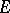in a spaceThe function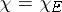that is equal to 1 when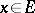and equal to 0 when(where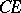is the complement toin). Every functiononwith values in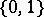is the characteristic function of some set, namely, the set. Properties of characteristic functions are:

1),;

2) if, then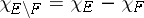;

3) if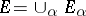, then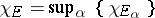;

4) if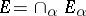, then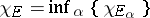;

5) ifare pairwise disjoint, then;

6) if, then.